# Hardest colleges to get into in Michigan

Top 10 colleges in Michigan with the lowest acceptance rates
What are the hardest colleges to get into in Michigan? We've got you covered. We've compiled a national college database and have created a list of the hardest universities to get into in Michigan below. These are the hardest 4 year colleges to get into in Michigan, and sometimes some of the hardest colleges to get into in the US. You could even say these are the best colleges in Michigan. We also include each college's average SAT and ACT scores so that you can see where you're most competitive. Read on to find out more.

## University of Michigan at Ann Arbor acceptance rate

University of Michigan at Ann Arbor acceptance rate is 22.9%.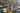The average SAT score for University of Michigan at Ann Arbor is 1435.

• SAT composite: 1435
• SAT math: 735

The average ACT score for University of Michigan at Ann Arbor is 33.

## Hillsdale College acceptance rate

Hillsdale College acceptance rate is 36%.The average SAT score for Hillsdale College is 1363.

• SAT composite: 1363
• SAT math: 670

The average ACT score for Hillsdale College is 31.

## Finlandia University acceptance rate

Finlandia University acceptance rate is 37.7%.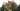The average SAT score for Finlandia University is 920.

• SAT composite: 920
• SAT math: 470

The average ACT score for Finlandia University is 21.

## College for Creative Studies acceptance rate

College for Creative Studies acceptance rate is 45.6%.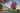The average SAT score for College for Creative Studies is 1110.

• SAT composite: 1110
• SAT math: 555

The average ACT score for College for Creative Studies is 22.

## Aquinas College acceptance rate

Aquinas College acceptance rate is 51.9%.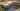The average SAT score for Aquinas College is 1250.

• SAT composite: 1250
• SAT math: 590

The average ACT score for Aquinas College is 24.

Adrian College acceptance rate is 56.3%.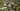The average SAT score for Adrian College is 1140.

• SAT composite: 1140
• SAT math: 570

The average ACT score for Adrian College is 23.

## Andrews University acceptance rate

Andrews University acceptance rate is 57.7%.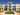The average SAT score for Andrews University is 1199.

• SAT composite: 1199
• SAT math: 600

The average ACT score for Andrews University is 25.

## Alma College acceptance rate

Alma College acceptance rate is 64%.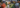The average SAT score for Alma College is 1134.

• SAT composite: 1134
• SAT math: 560

The average ACT score for Alma College is 23.

## University of Michigan-Flint acceptance rate

University of Michigan-Flint acceptance rate is 65.1%.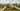The average SAT score for University of Michigan-Flint is 1081.

• SAT composite: 1081
• SAT math: 530

The average ACT score for University of Michigan-Flint is 23.

## Cornerstone University acceptance rate

Cornerstone University acceptance rate is 67.4%.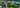The average SAT score for Cornerstone University is 1083.

• SAT composite: 1083
• SAT math: 510